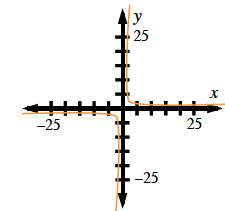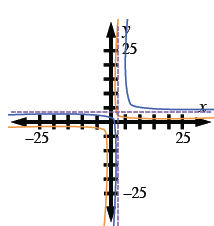### Home > AC > Chapter 13 > Lesson 13.CS1-S > Problem4-138

4-138.

Explain the difference between the graphs of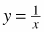and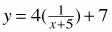. Homework Help ✎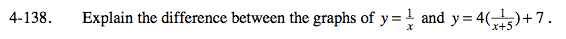Here is an example:

$\textit{y}=\frac{3}{\textit{x}}$

$\textit{y}=2\left(\frac{3}{\textit{x}-2}\right)+4$

The second equation is shifted 2 right and 4 up, and is stretched by a factor of 2.